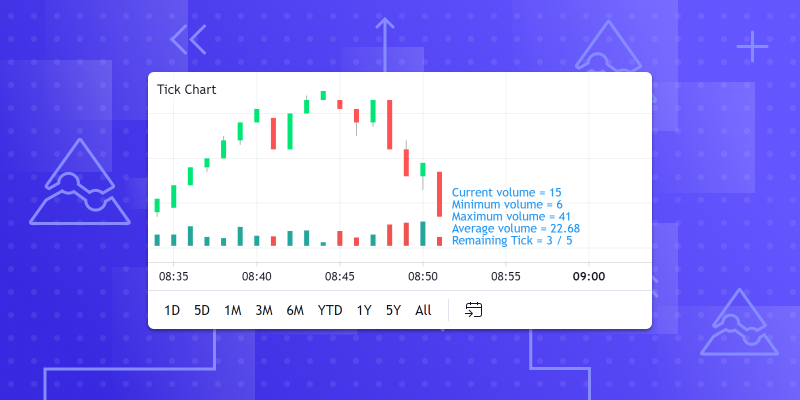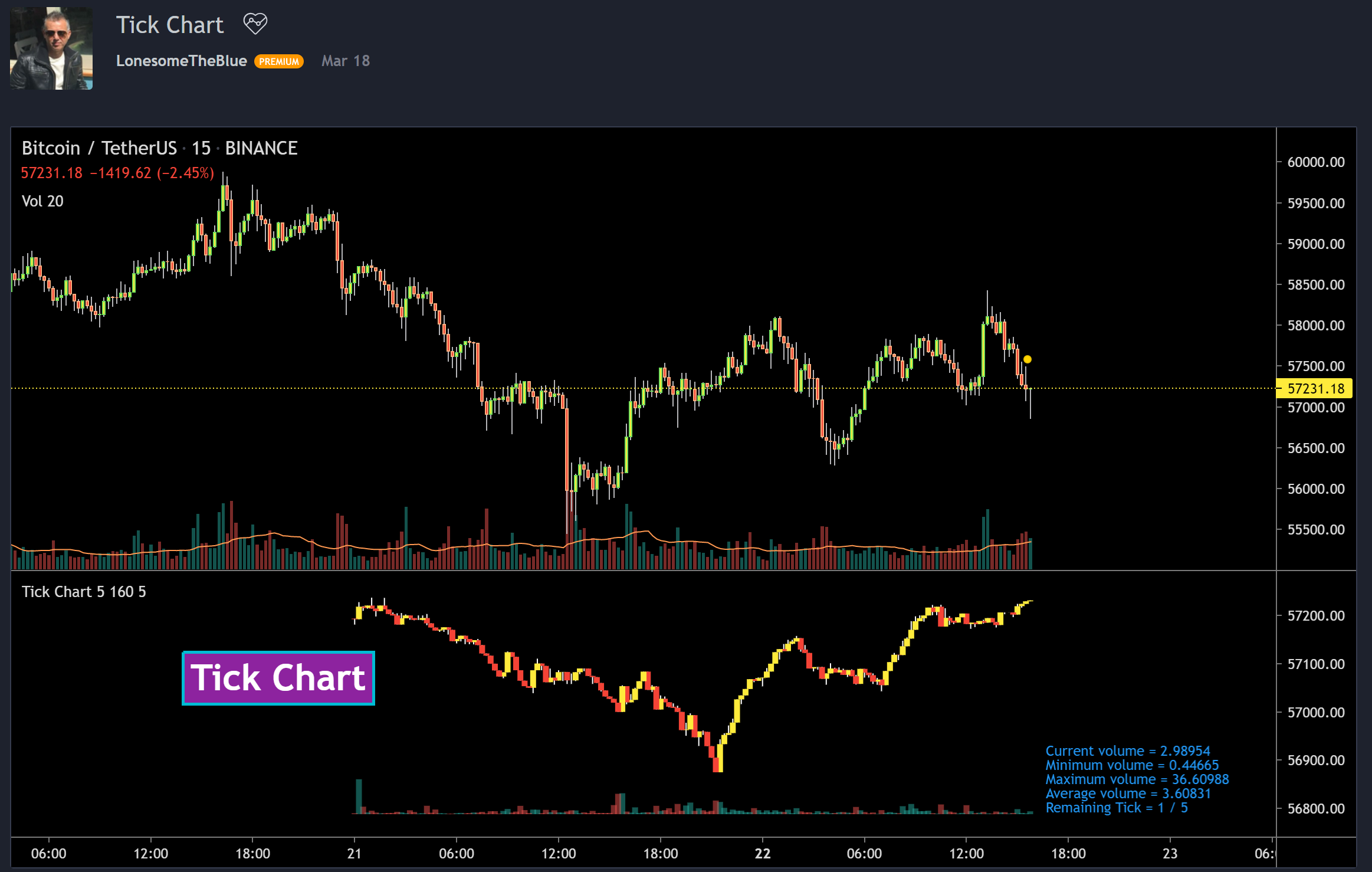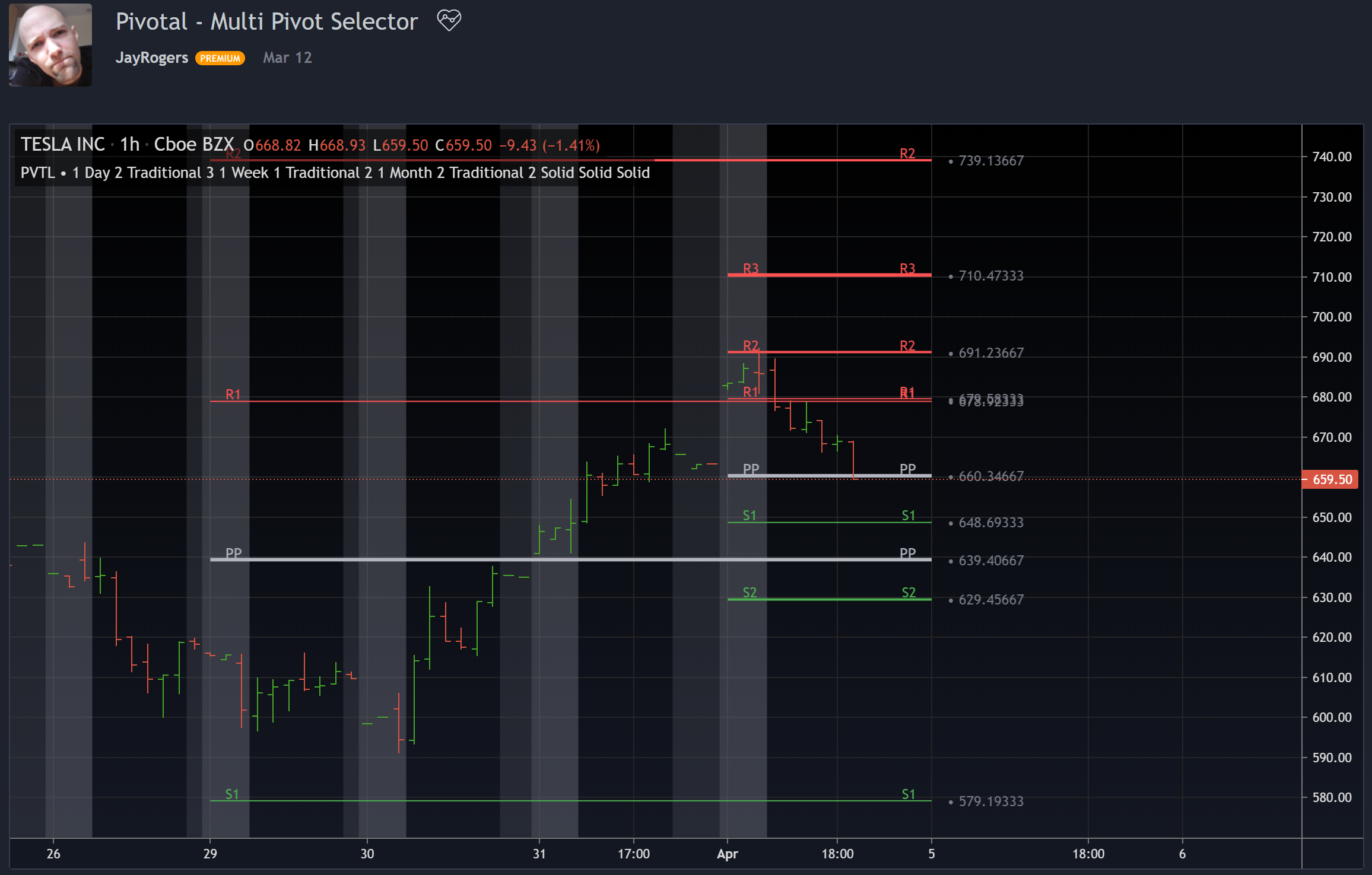April 9, 2021
Pine有什么新功能？• 新常数 math.pimath.phimath.rphi 和 math.e
• 新的 round() 功能 — 该函数现在允许您指定舍入的精度，而不必始终舍入为最接近的整数
• median()
• mode()
• range()
• todegrees()
• random()

```//@version=4
strategy("I’m feeling lucky", overlay=true)
seed = input(1, "Seed, increase to recalculate the strategy", minval=1)
entryPerc = input(20.0, "Entry chance, %", minval=0.0, maxval=100.0)
exitPerc = input(10.0, "Exit chance, %", minval=0.0, maxval=100.0)
roll = random(0, 100, seed)
positionSize = floor(random(1, (strategy.equity / close), seed))
if roll < entryPerc
strategy.entry("My Long Entry Id", strategy.long, qty=positionSize)
if roll < exitPerc
strategy.close_all()```

```//@version=4
study("My SMAs", overlay=true)
sma_lengths = input("5, 10, 20, 30, 50", title = "SMA Lengths (separated by comma)")
sma_lengths_array = str.split(str.replace_all(sma_lengths, " ", ""), ",")
sma_len(index) =>
index > array.size(sma_lengths_array) - 1 ? 10 :
int(tonumber(array.get(sma_lengths_array, index)))
sma0 = sma(close, sma_len(0))
sma1 = sma(close, sma_len(1))
sma2 = sma(close, sma_len(2))
sma3 = sma(close, sma_len(3))
sma4 = sma(close, sma_len(4))
plot(sma0, color=color.blue)
plot(sma1, color=color.red)
plot(sma2, color=color.green)
plot(sma3, color=color.orange)
plot(sma4, color=color.purple)``````//@version=4
study(title="Aroon", shorttitle="Aroon", overlay=false, format=format.percent, precision=2, resolution="")
length = input(14, minval=1)
upper = 100 * (highestbars(high, length+1) + length)/length
lower = 100 * (lowestbars(low, length+1) + length)/length
plot(upper, "Aroon Up", color=#FF6A00)
plot(lower, "Aroon Down", color=#0094FF)```

session.ismarketsession.ispremarket 和 session.ispostmarket 这三个新变量，使您可以检查日内交易时段与当前K线相关的部分。请注意，延长时段仅显示在日内图表上，并且仅在通过设置明确启用了该选项时才会显示。